# IBPS PO Prelims Reasoning Ability Questions 2019 (Day-11)

Dear Aspirants, Our IBPS Guide team is providing new series of Reasoning Questions for IBPS PO Prelims 2019 so the aspirants can practice it on a daily basis. These questions are framed by our skilled experts after understanding your needs thoroughly. Aspirants can practice these new series questions daily to familiarize with the exact exam pattern and make your preparation effective.

Check here for IBPS PO Prelims Mock Test 2019

[WpProQuiz 7076]

Directions (1-5): Study the following information carefully and answer the given question:

Eight persons Ram, Rahul, Raju, Sam, Sanjay, Sambhu, Joseph and Jack are sitting in circular table facing towards the centre. All the persons are sitting along the circumference of the circular table at an equal distance between them. The radius(r) of the circle is 70m and the circumference of the circle is 2πr, where π = 22/7. Distances are considered only on the circumference of the circular table.

Ram sits 110m away from Jack. Rahul sits second to the right of Sanjay who is not an immediate neighbour of Jack. Joseph sits 55m away from the right of Sanjay. Raju and Sam sit opposite to each other. Sam sits 110m to the left of Sambhu. Rahul is not an immediate neighbour of Ram. Ram doesn’t sits opposite to Rahul.

1) Who among the following sits 265m right of Sam?

a) Rahul

b) Raju

c) Sanjay

d) Jack

e) None of these

2) Who among the following sits second to the right of Sambhu?

a) Ram

b) Rahul

c) Raju

d) Sanjay

e) None of these

3) Which of the following statement(s) is/are correct?

i) Joseph and Sambhu are sitting opposite to each other

ii) Jack sits immediate left of Sambhu

a) Only (i)

b) Only (ii)

c) Both (i) and (ii) are correct

d) Data insufficient

e) Both (i) and (ii) are incorrect

4) Who sits immediate left of Rahul?

a) Sanjay

b) Joseph

c) Ram

d) Sam

e) None of these

5) If all the persons are made to sit in the alphabetical order in clockwise direction starting from Jack, then the position of how many persons will remain unchanged (including Jack), when compared to the original seating positions?

a) Two

b) Four

c) One

d) Three

e) More than four

Directions: (6-10): In the following question assuming the given statements to be true, find which of the conclusion among given five conclusions is/are definitely true and then give your answers accordingly.

6) Statement: B > K ≤ M; S < T ≥ R; M = N < R

Conclusion:

i) T > K

ii) S ≤ N

iii) B < S

a) only conclusion i follows

b) only conclusion ii follows

c) only conclusion i and iii follows

d) All i, ii and iii follows

e) None follows

7) Statement: J ≤ A < D ; M ≤ N < K = L ; B ≥ K ;  D > B

Conclusion:

i) J > K

ii) B ≤ M

iii) D > N

a) only conclusion i follows

b) only conclusion iii follows

c) only conclusion i and iii follows

d) All i, ii and iii follows

e) None follows

8) If Q ≤ E < H ; J ≥ V=O > Y ; J < I ≤ H, which of the following is definitely true?

a) Q < V

b) I < Y

c) H > Y

d) H ≥ O

e) None of these

9) Which of the following expression satisfies U < F, E > T as definitely true and Q < U as definitely false?

a) T < U ≤ C < Y = E ≥ F ≤ Q

b) E < F ≤ Q ≥ U = C > T < Y

c) U ≤ C = E < F ≤ Q > T > Y

d) Y > T< U ≤ C = E < F ≤ Q

e) None of these

10) Which of the following fits in the blank to make the expression S>J, Q≤O is definitely true respectively from left to right?

_____ ≤ _______ ≤ _____= ______ > _______

a) B, S, O, J, Q

b) S, O, B, Q, J

c) Q, B, S, O, J

d) J, Q, B, S, O

e) None of these

Direction (1-5):

• All the persons are sitting along the circumference of the circular table at an equal distance between them.
• The radius(r) of the circle is 70m and the circumference of the circle is 2πr, where π = 22/7.

Therefore circumference = 2*22/7 *70 = 440, and there are 8 persons sits in a table. So 440/8 = 55m which is the distance between two successive person.

• Distances are considered only on the circumference of the circular table.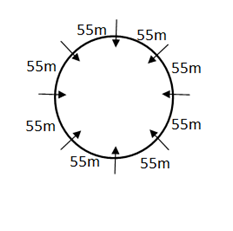• Ram sits 110m away from Jack. There are two possible cases, Either Ram sits 110m away from left or right of Jack.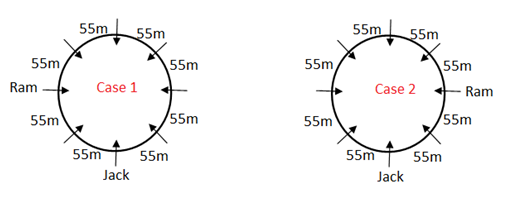• Rahul sits second to the right of Sanjay who is not an immediate neighbour of Jack.
• Rahul is not an immediate neighbour of Ram.
• For these above statements we have three possible cases for case 2 (case 2a, case 2b, case 2c).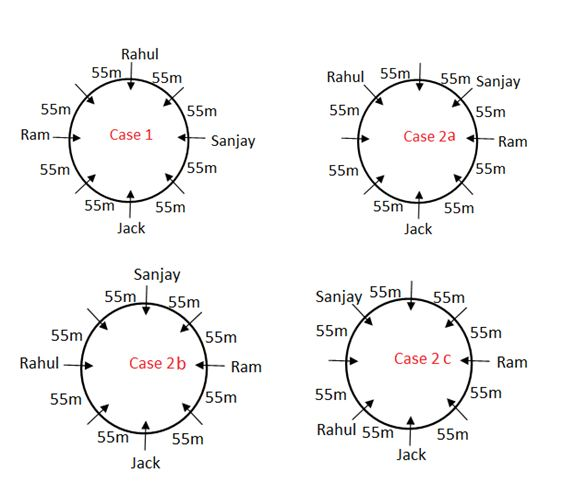• Joseph sits 55m away from the right of Sanjay.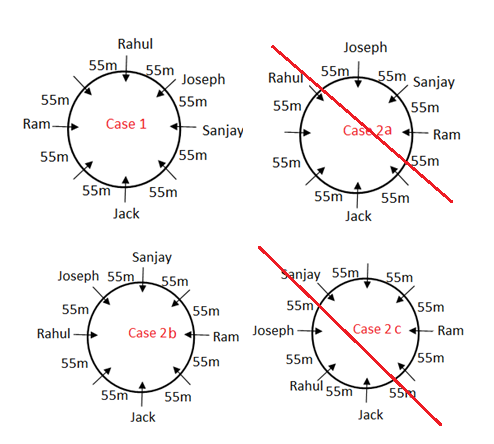• Raju and Sam sit opposite to each other.
• Sam sits 110m to the left of Sambhu.
• From the above statement we cannot fix the position for Raju and Sam in case 2a and case 2c. So this case is eliminated.
• Ram doesn’t sits opposite to Rahul.
• So, the Case 2b gets eliminated.
• And finally, we get the following arrangement.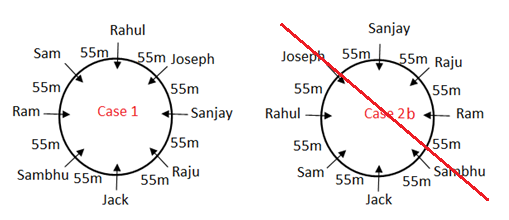Direction (6-10):

B < K ≤ M=N< R ≤ T > S

J ≤ A < D > B ≥ K > N ≥ M

Q ≤ E < H ≥ I > J ≥ V = O > Y